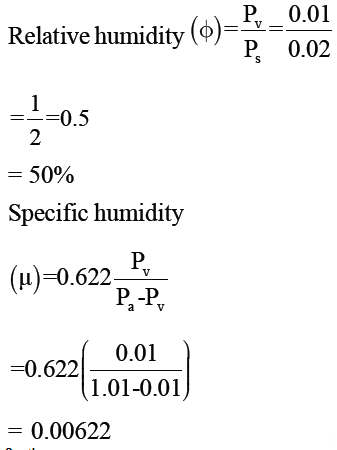Courses

# Refrigeration & Air Conditioning

## 20 Questions MCQ Test GATE Mechanical (ME) 2022 Mock Test Series | Refrigeration & Air Conditioning

Description
This mock test of Refrigeration & Air Conditioning for GATE helps you for every GATE entrance exam. This contains 20 Multiple Choice Questions for GATE Refrigeration & Air Conditioning (mcq) to study with solutions a complete question bank. The solved questions answers in this Refrigeration & Air Conditioning quiz give you a good mix of easy questions and tough questions. GATE students definitely take this Refrigeration & Air Conditioning exercise for a better result in the exam. You can find other Refrigeration & Air Conditioning extra questions, long questions & short questions for GATE on EduRev as well by searching above.
QUESTION: 1

### In a refrigerator refrigerant-134a enters the compressor as superheated vapor at a rate of 0.12 kg/s with h = 246.36 kJ/kg and it leaves with h = 288.53 kJ/kg. The isentropic enthalpy of refrigerant leaving compressor is hs = 281.16 kJ/kg. The refrigerant is cooled in the condenser and it leaves the condenser in subcooled region with h = 84.98 kJ/kg. It is then throttled to 0.15 MPa. The isentropic efficiency of compressor and COP of refrigerator respectively are

Solution: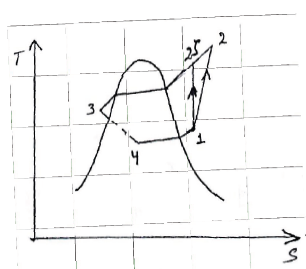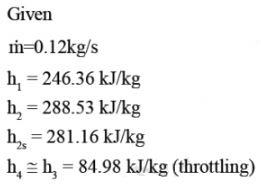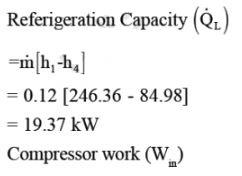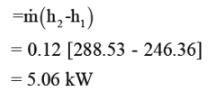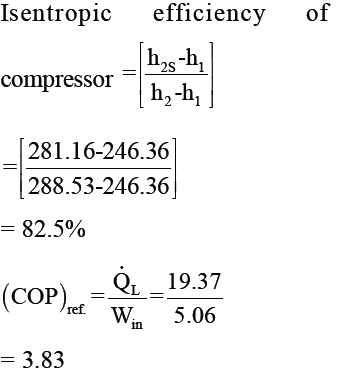QUESTION: 2

### In a certain cooling dehumidification operation 360 kg/h of air (DBT 350C & RH 50%) is blown over a cooling coil having apparatus dew point of 50C, assuming a bypass factor of 15%, the outlet temperature of air is

Solution: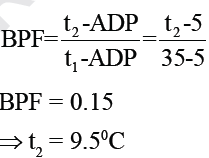QUESTION: 3

### An air refrigerator of 10 ton capacity operates in Bell coleman  cycle between pressure limits of 1bar and 4 bar. Air at inlet of compressor is at 1 bar and 253K. Air at exit of cooler is at 313 K. After air is cooled to 290K at constant pressure in cooler, it is allowed to expand isentropically to 1 bar in expansion cylinder. Cold air is then sent to cold chamber to extract heat (Refrigeration effect). Determine COP of refrigerator.

Solution: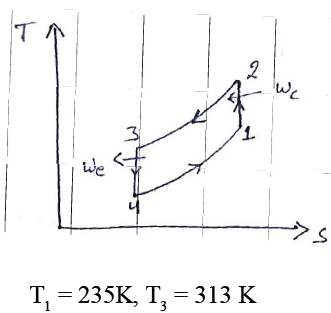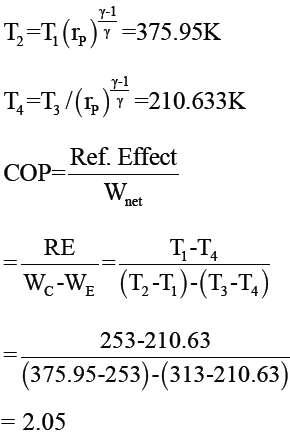QUESTION: 4

Under saturated ambient air conditions:
[DBT = Dry bulb temperature,
WBT = Web bulb temperature]

Solution:

For Saturated air
WBD = DBT - WBT = 0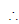DBT = WBT

QUESTION: 5

Common data question 5 & 6

A refrigerator operates on an ideal vapor - compression refrigration cycle between 0.14 MPa and 0.8 MPa. The mass flow rate of the refrigerant is 0.05 kg/s. Take hg (at 0.14 MPa) = 239.16 kJ/kg, Sg (at 0.14 MPa) = 0.94456 kJ/kgK, hf (at 0.8 MPa) = 95.47 kJ/kg and h (at compressor exit) = 275.39 kJ/kg

Q. The rate of heat removal from refrigerated space and the heat rejected to the atmosphere respectively are

Solution: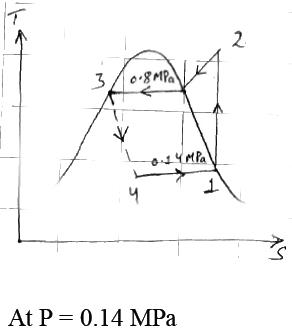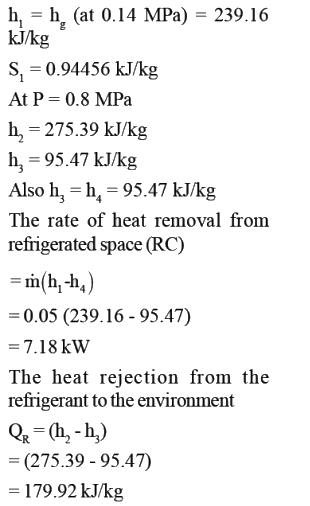QUESTION: 6

Common data question 5 & 6

A refrigerator operates on an ideal vapor - compression refrigration cycle between 0.14 MPa and 0.8 MPa. The mass flow rate of the  refrigerant is 0.05 kg/s. Take hg (at 0.14 MPa) = 239.16 kJ/kg, Sg (at 0.14 MPa) = 0.94456 kJ/kgK, hf (at  0.8 MPa) = 95.47 kJ/kg and h (at compressor exit) = 275.39 kJ/kg

Q. The COP of the refrigerator is

Solution: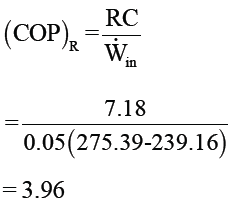QUESTION: 7

Horizontal lines moving towards the saturation curve on the psychrometric chart represents

Solution:
QUESTION: 8

Common Data Question 8 & 9

In a Carnot vapor refrigeration cycle refrigerant R - 134a is used as the working fluid. The refrigerant enters the condenser as saturated vapor at 280C and leaves as saturated liquid. The evaporator operates at a temperature of -100C. The thermodynamic properties for the refrigerant are given below.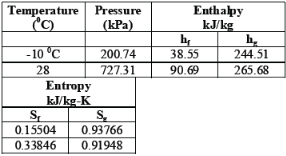Q. The net work for the cycle is given by

Solution: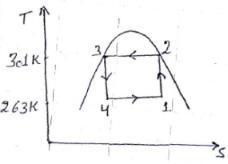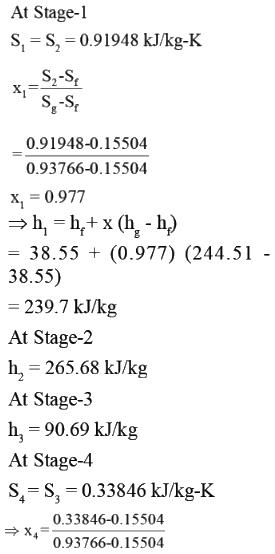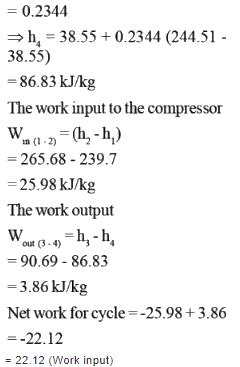QUESTION: 9

Common Data Question 8 & 9
In a Carnot vapor refrigeration cycle refrigerant R - 134a is used as the working fluid. The refrigerant enters the condenser as saturated vapor at 280C and leaves as  saturated liquid. The evaporator  operates at a temperature of -100C. The thermodynamic properties for the refrigerant are given below.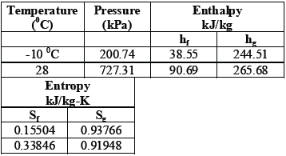Q. The coefficient of performance of the cycle is

Solution: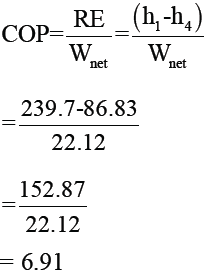QUESTION: 10

The dry-bulb, wet bulb and dew point temperatures are identical at

Solution: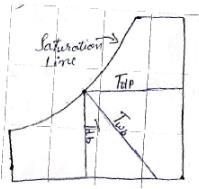For the saturation air (100% ralative humidity), the dry bulb, wet bulb and dew point temperature identical.

QUESTION: 11

A room is filled with saturated moist air at 250C and a total pressure of 100 kPa. The saturated pressure at 250C is 3.1698 kPa. If the mass of dry air in the room is 100 kg, what is the mass of water vapour?

Solution: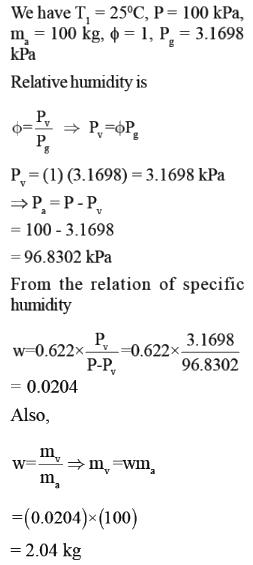QUESTION: 12

Common data for 12 & 13

A commercial refrigerator is used to keep the refrigerated space at -300C by rejecting its waste heat to cooling water. This cooling water enters the condenser at 180C (hw = 75.47 kJ/kg) with a rate of 0.25 kg/s and leaves at 260C (hw = 108.94 kJ/kg). The refrigerant enters the condenser at 1.2 MPa, 650C (h = 295.16 kJ/kg) and leaves at 420C (h = 111.23 kJ/kg). The inlet state of the compressor is 60 kPa, -340C (h = 230.03 kJ/kg) and the compressor is estimated to gain a net heat of 450W from the surroundings:

Q. The refrigeration load will be

Solution: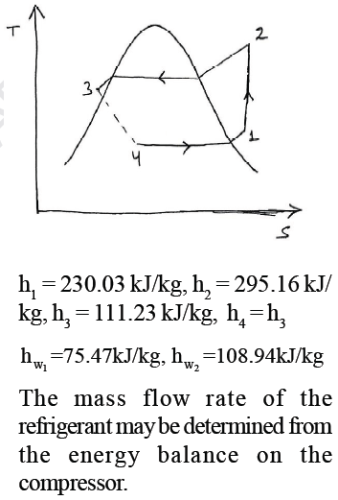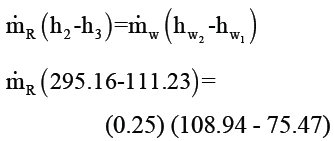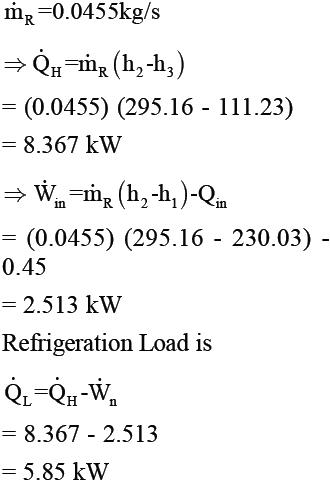QUESTION: 13

Common data for 12 & 13

A commercial refrigerator is used to keep the refrigerated space at -300C by rejecting its waste heat to cooling water. This cooling water enters the condenser at 180C (hw = 75.47 kJ/kg) with a rate of 0.25 kg/s and leaves at 260C (hw = 108.94 kJ/kg). The refrigerant enters the condenser at 1.2 MPa, 650C (h = 295.16 kJ/kg) and leaves at 420C (h = 111.23 kJ/kg). The inlet state of the compressor is 60 kPa, -340C (h = 230.03 kJ/kg) and the compressor is estimated to gain a net heat of 450W from the surroundings:

Q. The theoretical maximum refrigeration load for the same power input to the compressor is

Solution: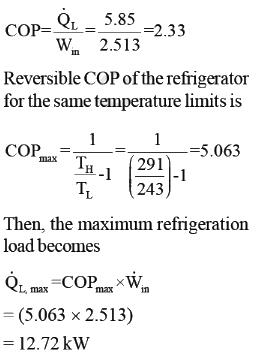*Answer can only contain numeric values
QUESTION: 14

A heat pump operates between the temperature of 320C and -100C. The rates of heat addition and heat rejection are 650W and 1000 W respectively. The COP for the heat pump is ____

(Important - Enter only the numerical value in the answer)

Solution: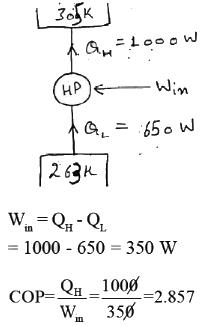QUESTION: 15

In a VARs heating in generator is done at 2000C, refrigeration in evaporator at -50C and cooling in condenser at 270C. The maximum COP of the system is

Solution: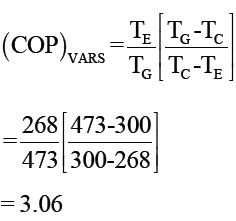*Answer can only contain numeric values
QUESTION: 16

In an ideal VCRS, the enthalpy of the refrigerant before and after the evaporator are 90 kJ/kg and 200 kJ/kg. The circulation rate of refrigerant for each tonn of refrigerant is_____kg/min.

(Important - Enter only the numerical value in the answer)

Solution: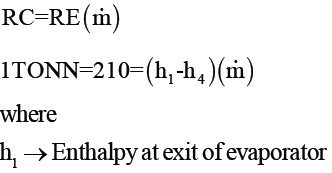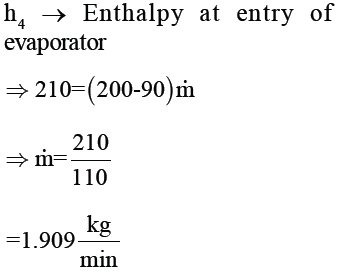QUESTION: 17

The chemical formula for the refrigerant R-22 is given by

Solution: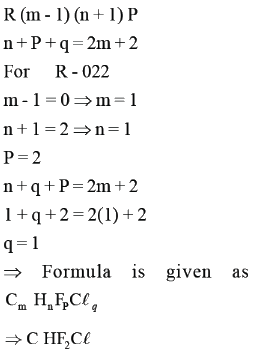QUESTION: 18

The efficiency of Carnot engine is given as 0.80. If the cycle direction is reversed, the COP of the Carnot
refrigerator is_____

(Important - Enter only the numerical value in the answer)

Solution: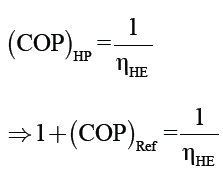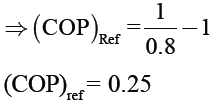*Answer can only contain numeric values
QUESTION: 19

Organic material of 1800 kg is to be stored in refrigerator storage. The material is supplied at 270C and is to be cooled to -300C for preserving it. The cooling takes place in 12 hours. The specific heat of material is 2 kJ/kgK above freezing point and 0.6 kJ/kg-K below freezing point, which is -30C. The latent heat of freezing is 230  kJ/kg. If the actual COP is half of the ideal COP, the power required to drive the plant is ____ kW.

(Important - Enter only the numerical value in the answer)

Solution: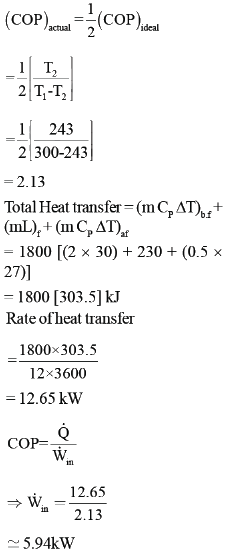QUESTION: 20

Moist air exists at a pressure of 1.01 bar. The partial pressure and saturation pressure of water vapour are 0.01 bar and 0.02 bar respectively. The relative humidity and humidity ratio of the moist air, respectively are

Solution: